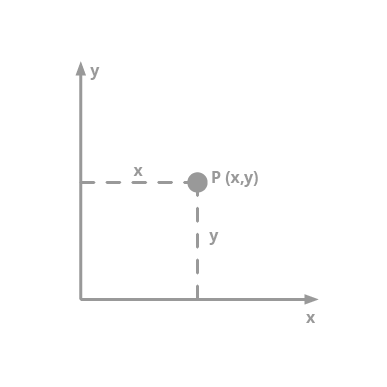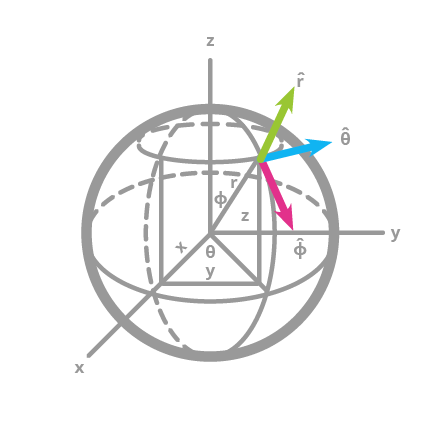Deals Of The Week - hours only!Up to 80% off on all courses and bundles.-Close
Introduction
Geometry vs. Geography
Summary

## Instruction

Essentially, geometry columns represent 2D figures. They use the Cartesian coordinate system which you may remember from school. Calculations in this system are relatively easy: finding the area of a polygon or a distance between two points involves simple math.However, in Part 2, we said that it is impossible to find an ideal projection of the Earth (which is a sphere) onto a 2D plane. That is why calculations related to the Earth are not very accurate when we use geometry columns.

Geography columns are a different story. They are called geography because they are related to geography, or the globe, strictly speaking. In geography columns, spherical coordinates are used instead to describe points. A point is defined by an angle of rotation from a reference meridian (the Prime Meridian), and the angle from the equator. These two angles are longitude and latitude, respectively. As you can see, geography columns rely on much more complex math. Though geography columns are very accurate, they are also much slower than geometry columns.## Exercise

Click to continue.